# How Many Squares in a Checkerboard?

Last week we looked at ways to count paths along the edges of a rectangular grid. Now we’ll look at a companion problem: counting the number of squares (or rectangles) of all sizes in a square (or rectangular) grid. This, too, is a very common question, and I’ll be picking just a few of many answers we’ve given to variants of the problem.

## Squares in an 8×8 or n×n board

We’ll start with a question from the very first week Ask Dr. Math was in operation, in November 1994:

Chessboard Squares

Dear Dr. Math,

We are students from Sherwood Elementary in Edmonds, WA.  We have been working on problems in which we investigate patterns and functions.  We worked on a problem called the chessboard squares.  We discovered that there are 204 squares on the board and we found several ways to look at it.  We found that you would add the different squares - 1 + 4 + 9 + 16 + 25 + 36 + 49 + 64.  We did this and it worked because of the pattern, but our teacher wants to know why it works and if there is a quick way to get to the answer.  What if there was a 100 by 100 board?  Is there a way to use a function to find this out?

We would love to hear from you.
6th grade students in Mrs. Moore's room.

To clarify the question, here are all the $$1+4+9=14$$ squares in a 3×3 grid: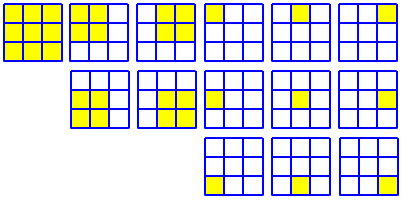Hello there!

I think I figured out what you mean, and I think it's a fantastic question! You mean the number of squares of any size, right? So in the above sum, the 1 corresponds to the number of 8x8 squares, the 4 is the number of 7x7 squares, and so on, right? Let us know if it's not.

I won’t try to show all 204, but for example here are the 4 7×7 squares: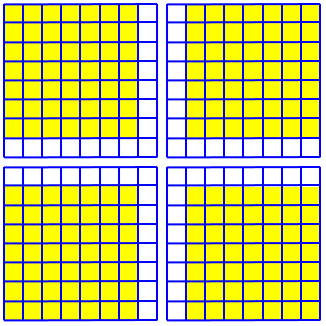Here's a clue about why it works. Picture the chessboard tacked up on a wall. Then let's say you have a 5x5 square sitting in the lower left corner. Where else can you slide that square to?  Well, you could keep it there, or you could slide it one space to the right, or two spaces to the right, or three spaces to the right; or you could slide it up zero, up one, up two, or up three spaces. Also, you could do both, and slide it up one and over two, or any of the various combinations of slidings.

Here are the 16 ways you could slide it (including leaving it alone!):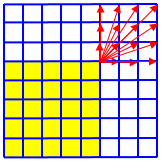They correspond to the 16 places the upper right corner could be located.

So there are four options for sliding in each of the two directions, right? Zero spaces, one spaces, two spaces, or three. So the number of different places you could put that 5 by 5 square is 4 times 4 (4 in each of the two directions).

How many different places could you put a 4 by 4 square on the board?  Can you come up with a formula, so that if I tell you the size of the square (like five by five, or four by four), you could tell me the number of different places you could put it in the chessboard?

What we find is that for a k×k square in an n×n board, there are $$(n-k+1)$$ possible positions in each direction, for a total of $$(n-k+1)^2$$ such squares. For the total, we just have to add these together. This could be written as $$\sum_{k=1}^n (n-k+1)^2 = n^2+(n-1)^2+\cdots+1^2$$ but since the numbers being squared range from $$n$$ down to 1, this can more nicely be written as $$\sum_{k=1}^n k^2 = 1^2+2^2+\cdots n^2$$

### Making a formula

The second part of your question is also very interesting.  I'm very impressed that you're doing it in elementary school;  I didn't see that problem until high school!

You want to know a formula for the sum of the first n perfect squares. Well, it's kind of tricky to derive, but I do happen to know what it is. Ordinarily, I wouldn't just give it to you without some kind of proof or derivation, or at least some hint of what's actually going on, but the only proof I know is, I think, too sophisticated for elementary school (If I'm wrong, PLEASE let us know!  I don't want to underestimate your capabilities!).  The proof uses a concept called mathematical induction.

Anyway, the formula for the sum of the first n perfect squares is

n x (n + 1) x (2n + 1)
______________________
6

Check it out to make sure that it works.  If you have any more questions, send them on in!

We have discussed this formula many other times; next week I’ll show several proofs, as well as ways to discover this formula (which mathematical induction doesn’t do for us). We’ll start, in fact, with a response to a question about this very answer, which was later attached to the same page.

## Counting squares again …

Next, we have a 1997 question, even more specifically asking for a simple formula:

Checkerboard Squares

My teacher gives us a problem of the week and I haven't really been able to figure out this last one. Here's the question:

Say you have an 8x8 checkerboard. There are many other little and big squares inside of that so counting them all how many are there? (e.g. counting all the 1x1,2x2,3x3,4x4,5x5,6x6,7x7,8x8).

Suppose you have a square checkerboard of some other size - not 8x8 - what is the easiest way to find how many squares there are in it?

Make sure that when you are done you will be able to find the number of squares in any size checkerboard within 2 minutes.

I tried counting the squares individually, but that wouldn't be quick enough for 12x12. Could you please give me some tips for how to approach this problem?

Thanks,
Tash

Doctor Anthony answered, starting this time with the smallest square:

Consider the left hand vertical edge of a square of size 1 x 1.  This edge can be in any one of 8 positions. Similarly, the top edge can occupy any one of 8 positions for a 1 x 1 square. So the total number of 1 x 1 squares = 8 x 8 = 64.

For a 2 x 2 square the left hand edge can occupy 7 positions and the top edge 7 positions, giving 7 x 7 = 49 squares of size 2 x 2.

Continuing in this way we get squares of size 3 x 3, 4 x 4 and so on.
We can summarize the results as follows:

Size Of square      Number of squares
---------------     -----------------
1 x 1               8^2 = 64
2 x 2               7^2 = 49
3 x 3               6^2 = 36
4 x 4               5^2 = 25
5 x 5               4^2 = 16
6 x 6               3^2 =  9
7 x 7               2^2 =  4
8 x 8               1^2 =  1
---------------
Total   = 204

(This, without a closed-form formula, would be good enough to give an answer for a 12×12 board within two minutes; but the formula will be easier for really large boards!)

Then he gave the formula, again with no proof:

There is a formula for the sum of squares of the integers 1^2 + 2^2 + 3^2 + ...  + n^2

n(n+1)(2n+1)
Sum  = ------------
6

In our case, with n = 8, this formula gives  8 x 9 x 17/6 = 204.

Again, we’ll get to the proof next week.

### … and counting rectangles

But then he suggested a different problem, one that leads very easily to a formula:

As an extension to this problem, you might want to calculate the number of rectangles that can be drawn on a chessboard.

There are 9 vertical lines and 9 horizontal lines. To form a rectangle you must choose 2 of the 9 vertical lines, and 2 of the 9 horizontal lines. Each of these can be done in 9C2 ways = 36 ways. So the number of rectangles is given by 36^2 = 1296.

Here is an example: In our 8×8 chessboard, label the vertical lines 1 through 9, and likewise the horizontal lines. Any choice of two pairs, such as (2, 5) and (3, 7) here, defines a rectangle: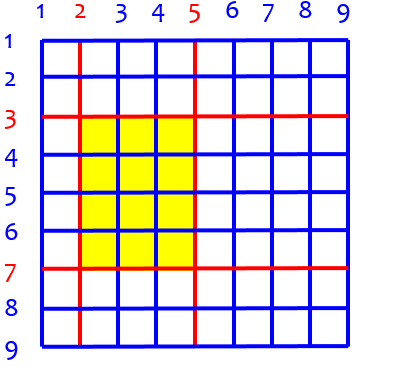There are $${9\choose 2}=\frac{9\cdot8}{2\cdot1} = 36$$ ways to choose each pair, for a total of $$36\cdot 36=1296$$ possible rectangles. (This is, of course, far more than the number of squares, which is a subset of the rectangles.)

The same can be done to count rectangles in a general m×n rectangle. The result is $${{m+1}\choose 2}{{n+1}\choose 2} = \frac{(m+1)m}{2}\frac{(n+1)n}{2} = \frac{mn(m+1)(n+1)}{4}$$ For example, in a 2×3 rectangle, there are $$\frac{2\cdot 3(2+1)(3+1)}{4} = 18$$ rectangles (remember that a square is a kind of rectangle):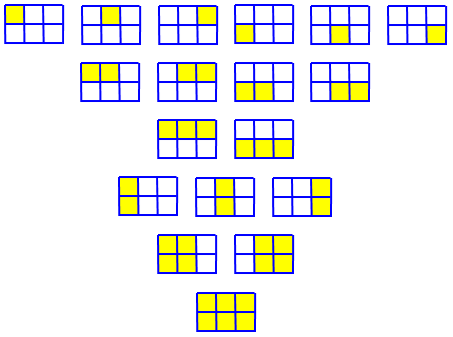## Squares in an m×n rectangle: getting started

Now let’s move on to a harder problem: What if the whole grid is a rectangle rather than a square, but we’re still counting squares? Here is a question from 2003:

Formula for Squares inside Rectangles

Is there an equation for how many squares there are in a rectangle divided up into 1cm blocks? This is like the typical chessboard puzzle.

Hi Jamie,

There is a formula that will calculate the number of squares in a rectangle. Here is how I found it.

First, let's consider only rectangles whose width is 1.

A 1x1 rectangle contains exactly one square, a 1x2 rectangle contains two squares, a 1x3 rectangle contains three squares, etc.  In general, if the dimensions of a rectangle are 1xn, there will be n squares.

That was easy, and I probably haven't told you anything you didn't already know.

This is trivial; here are the 5 squares in a 1×5 rectangle: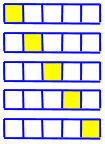But starting with an easy case is a classic approach to problem solving, and we can build on this:

Now let's consider rectangles whose width is 2, starting with a 2x2 rectangle. We skip the 2x1 rectangle because we've already considered that in the previous case.  A 2x2 rectangle consists of four squares whose dimensions are 1x1 and one square whose dimensions are 2 x 2.  This gives us a total of five squares.

I'm going to create a table in which I list the width, length, number of 1x1 squares, number of 2x2 squares, and total number of squares.

W x L    1x1  2x2  total
-----    ---  ---  -----
2 x 2     4    1     5
2 x 3     6    2     8
2 x 4     8    3    11
2 x 5    10    4    14

In general, you should see that if you have a 2xn rectangle, the total number of squares will be 3n - 1.

Here are the 14 squares in a 2×5 rectangle: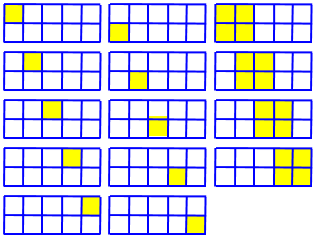He has simply recognized an apparent pattern: The total increases by 3 when the length increases by 1. Can you see a reason to believe this is always true? Hint: Here, in red, are the 3 squares that are added when we extend from 2×4 to 2×5: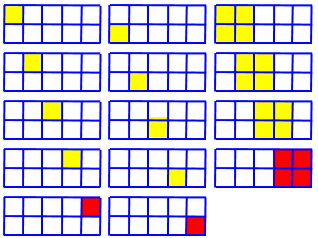I repeated this process for 3xn rectangles, 4xn rectangles, 5xn rectangles, until I recognized that there was a pattern developing.  If the width is m and the length is n, I was able to write the total number of squares in terms of m and n.

Give it a try and if you want to check your answer with me or if you have difficulties or other questions, write back to me and I'll try to help you some more.

Take some time to play with this before reading on.

## Squares in an m×n rectangle: a formula

Jamie wrote again the next day; as it was not a reply, it was treated as a new question (and the two were never connected!):

Squares in Rectangle Formula

What is the equation for the number of squares in a rectangle (like the chessboard puzzle)?

I have worked out that if the width is 2, then the formula for 2xn is equal to 3n-1. Is there an equation for a rectangle with a width of three? If so, how do you work it out and what is it?

I have made a chart but can see no relationship.

dimensions  1x1  2x2  3x3  total
3x2          6    2    0     8
3x3          9    4    1    10
3x4         12    6    2    20

This is essentially what Doctor Jaffee had done; Jamie has just made the next table. (Do you see an error in the table? That may be why Jamie didn’t see a pattern!)

Doctor Anthony answered this time, and (as he often did) went all the way to a formula:

In the general case we have an n x m rectangular board.

Consider the left-hand vertical edge of a square of size 1 x 1. This edge can be in any one of n positions. Similarly the top edge can occupy any one of m positions for a 1 x 1 square. So the total number of 1 x 1 squares = n x m.

Again, this is the obvious case; but it starts a process. We’ll be counting the number of locations for a square of a given size, rather than increasing the size of the board step by step:

For a 2 x 2 square the left-hand edge can occupy (n-1) positions and the top edge (m-1) positions, giving (n-1)(m-1) squares of size 2 x 2.

Continuing in this way we get squares of size 3 x 3, 4 x 4 and so on.

If we have an 8 x 9 board the numbers of squares are as follows:

Size of Square        Number of Squares
--------------        -----------------
1 x 1                  8 x 9  = 72
2 x 2                  7 x 8  = 56
3 x 3                  6 x 7  = 42
4 x 4                  5 x 6  = 30
5 x 5                  4 x 5  = 20
6 x 6                  3 x 4  = 12
7 x 7                  2 x 3  =  6
8 x 8                  1 x 2  =  2
----------------------------------------
Total  = 240

Now the question is, can we turn this sum into a simple formula?

We’ll assume that $$m>n$$, and let t be the difference between the two; then we can write the summation and use algebra to simplify the result:

For the general case of an  n x m  board, where m = n + t

n                n
We require  SUM[r(r + t)]  = SUM[r^2 + rt}
r=1              r=1

= n(n + 1)(2n + 1)/6 + tn(n + 1)/2

= [n(n + 1)/6]*[2n + 1 + 3t]

No. of squares = [n(n + 1)/6]*[2n + 3t + 1]  .......(1)

We’ll be breaking this down a little more soon, because he made a couple big leaps here.

As a check, we can try the formula on the example we added up manually above:

In the example above t = 1 and so

No. of squares  = 8 x 9/6[16 + 3 + 1]

= (72/6)

= 240    (as required)

This gives us reason to trust the formula.

The general formula for an (n x n+t) board is that given in (1) above.

No. of squares = [n(n + 1)/6]*[2n + 3t + 1]

$$\text{number of squares} = \frac{n(n+1)(2n+3t+1)}{6}$$

We can also rewrite this in terms of our original $$m = n + t$$, which yields $$\text{number of squares} = \frac{n(n+1)(2n+3(m-n)+1)}{6} = \frac{n(n+1)(3m-n+1)}{6}$$

A couple more examples:

Example(1): How many squares are there in 20 x 30 rectangular board?

Here n = 20 and t = 10

Number of squares = 20 x 21 x (1/6) x [40 + 30 + 1]

=  (1/6) x 20 x 21 x 71

=  4970

Example(2): How many squares are there in a 31 x 42 rectangular board?

Here n = 31 and t = 11

Number of squares = 31 x 32 x (1/6) x [62 + 33 + 1]

= (1/6) x 31 x 32 x 96

=  15872

He closed with a review of the facts needed for the derivation:

For the moment I think you should simply remember the formula

Number of squares = (1/6)*n(n + 1)(2n + 3t + 1)

where the rectangle has sides n and n + t.

The derivation depends on knowing the formulae for the sums of series like

1^2 + 2^2 + 3^2 + 4^2 + ... + n^2  = n(n+1)(2n+1)/6

and

1 + 2 + 3 + 4 + ... + n  = n(n+1)/2

You will probably be covering this topic in lessons before long and the formula I used for the number of squares in a rectangle will then become clear.

If you want to see the derivation of the sums of the series above you can write in again, but at the moment you may find the work difficult to follow.

### Doing it again, more visually

I still don't get how you came to find the rules as:

Squares in Rectangles:  n(n + 1)/6*[2n + 3t + 1]
Squares in Squares:  (n(n + 1)(2n + 1))/6

I don't understand where you have gotten the 6 from and how to section the equation into brackets.  Is there some way that you can point me in the right direction to coming to these conclusions?

Doctor Greenie derived the formula using an approach that parallels Doctor Anthony’s but is less algebraic:

Hi, Brooke -

I found Doctor Anthony's explanation of the problem on that page quite good -- but then, I already understand the problem.  I think there are a couple of things that could be done to improve his presentation of the material on that page.

The key to his method of deriving the formula (which is the method I find easiest to understand) is to consider the rectangle as a square array of squares with "extra" columns of squares added on.

Here is how he would think of a 3×5 rectangle: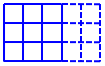With this approach, we can find

(1) The number of squares in an n-by-n square is

1^2 + 2^2 + 3^2 + ... + n^2

This sum is given by the formula

n(n + 1)(2n + 1)/6

Again, we’ll be looking at derivations of this formula next week.

(2) When we start with this n-by-n square and add 1-by-n columns of squares, the number of additional squares in the overall array resulting from the addition of each new column is

1 + 2 + 3 + ... + n

This sum is given by the formula

n(n + 1)/2

For example, adding the fourth column to our square adds these six squares: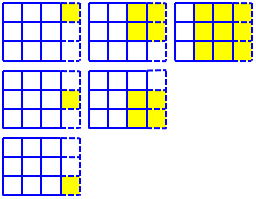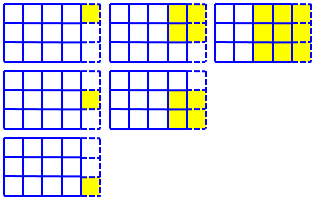So each column adds this same number of squares (which, you may notice, is the nth triangular number).

So, for example, the total number of squares in a 10-by-6 array of squares is the number of squares in the square 6-by-6 array, plus 4 (that is, 10-6) times the number of squares added with each additional column of 6 squares.  Doctor Anthony uses "n" as the dimension of the square array of squares and "t" as the number of additional columns, so the total number of squares in this case is given by the formula

n(n + 1)(2n + 1)/6 + (t)*[n(n + 1)/2]

With the specific case of a 10-by-6 array (n=6, t=4), this formula gives the total number of squares as

6(7)(13)/6 + 4(6)(7)/2 = 91 + 84 = 175

This formula is the same thing Doctor Anthony showed as the sum of a sum of squares and a sum of linear terms.

The algebra that followed deserves to be slowed down a bit:

Perhaps the most confusing part of Doctor Anthony's response (in my mind, at least) is that he disguises the formula above by combining the two terms into a single fraction with a common denominator.  To do that, the second fraction is multiplied by 3 in the numerator and denominator (to get the common denominator "6"), the two fractions are combined into a single fraction, and the common factors "n" and "(n+1)" in the numerator are factored out.  The steps are:

n(n+1)(2n+1)   (t)(n)(n+1)
------------ + -----------
6              2

n(n+1)(2n+1)   3(t)(n)(n+1)
------------ + ------------
6              6

n(n+1)(2n+1) + 3(t)(n)(n+1)
---------------------------
6

[n(n+1)]*[(2n+1) + 3t]
----------------------
6

n(n+1)(2n + 1 + 3t)
-------------------
6

n(n + 1)(2n + 3t + 1)
---------------------
6

n(n + 1)(2n + 3t + 1)/6

I much prefer the formula in the original form, because in that form you can see how the formula was obtained.

At the end, he gave links for a couple derivations of the formula for the sum of consecutive squares, which is the other part of what Brooke was asking for. We’ll look at those next time.

### 1 thought on “How Many Squares in a Checkerboard?”

This site uses Akismet to reduce spam. Learn how your comment data is processed.